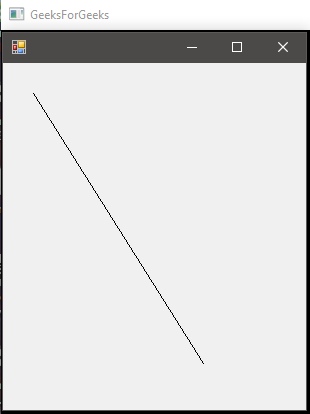Related Articles

# C# | Graphics.DrawLine() Method | Set – 2

• Last Updated : 01 May, 2019

Graphics.DrawLine() Method is used to draw a line connecting the two points specified by the coordinate pairs. There are 4 methods in the overload list of this method as follows:

• DrawLine(Pen, PointF, PointF) Method
• DrawLine(Pen, Int32, Int32, Int32, Int32) Method
• DrawLine(Pen, Single, Single, Single, Single) Method
• DrawLine(Pen, Point, Point) Method

First, two methods are already discussed in Set – 1. Here, we will discuss the last two methods.

#### DrawLine(Pen, Single, Single, Single, Single) Method

This method is used to draw line form a specified set of coordinates given in form x1, y1, x2, y2 all discrete.

Syntax:

public void DrawLine (System.Drawing.Pen pen, float x1, float y1, float x2, float y2);

Parameters:

• pen: Pen determines the color, width, and style of the line.
• x1: The abscissa of first point.
• y1: The ordinate of first point.
• x2: The abscissa of second point.
• y2: The ordinate of second point.

Exception: This method will give ArgumentNullException if the pen is null.

Example:

 `// C# program to illustrate the use of ``using` `System;``using` `System.Drawing;``using` `System.Drawing.Printing;``using` `System.Windows.Forms;`` ` `namespace` `GFG {`` ` `class` `PrintableForm : Form {`` ` `    ``// Main Method``    ``public` `static` `void` `Main()``    ``{``        ``Application.Run(``new` `PrintableForm());``    ``}`` ` `    ``public` `PrintableForm()``    ``{``        ``ResizeRedraw = ``true``;``    ``}`` ` `    ``protected` `override` `void` `OnPaint(PaintEventArgs pea)``    ``{``        ``// Defines the Pen``        ``Pen pen = ``new` `Pen(ForeColor);`` ` `        ``// x1 = 30``        ``// y1 = 30``        ``// x2 = 200``        ``// y2 = 300``         ` `        ``// using the Method``        ``pea.Graphics.DrawLine(pen, 30.0F, 30.0F, 200.0f, 300.0f);``    ``}``}``}`

Output:#### DrawLine(Pen, Point, Point)

This method is used to draw a line from a specified set of points to a specified set of points. It needs a Point variable which is set of (x, y) points.

Syntax:

public void DrawLine (System.Drawing.Pen pen, System.Drawing.PointF pt1, System.Drawing.PointF pt2);

Parameters:

• pen: Pen determines the color, width, and style of the line.
• pt1: Defines the (x, y) coordinates as a Point variable of the initial point.
• pt2: Defines the (x, y) coordinates as a Point variable of the final point.

Exception: This method will give ArgumentNullException if the pen is null.

Example:

 `// C# program to demonstrate the use of``// DrawLine(Pen, Point, Point) Method``using` `System;``using` `System.Drawing;``using` `System.Drawing.Printing;``using` `System.Windows.Forms;`` ` `namespace` `GFG {`` ` `class` `PrintableForm : Form {`` ` `    ``// Main Method``    ``public` `static` `void` `Main()``    ``{``        ``Application.Run(``new` `PrintableForm());``    ``}`` ` `    ``public` `PrintableForm()``    ``{``        ``ResizeRedraw = ``true``;``    ``}`` ` `    ``protected` `override` `void` `OnPaint(PaintEventArgs pea)``    ``{``        ``// Defines pen``        ``Pen pen = ``new` `Pen(ForeColor);`` ` `        ``// Defines the both points to connect``        ``// pt1 is (30, 30) which represents (x1, y1)``        ``Point pt1 = ``new` `Point(30, 30);`` ` `        ``// pt1 is (200, 300) which represents (x2, y2)``        ``Point pt2 = ``new` `Point(200, 300);`` ` `        ``// Draws the line``        ``pea.Graphics.DrawLine(pen, pt1, pt2);``    ``}``}``}`

Output:My Personal Notes arrow_drop_up